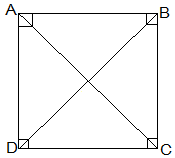Question

# $ABCD$ is a rectangle in which diagonal $AC$ bisects $\angle A$ as well as$\angle C$. Show that: $ABCD$ is a square.Diagonal $BD$ bisects $\angle B$ as well as $\angle D$.

Open in App
Solution

## Step $1:$ Drawing the diagram:$ABCD$ is a rectangle in which diagonal $AC$ bisects $\angle A$ as well as$\angle C$.Join diagonal $BD$.Step $2:$ Proving $ABCD$ is a square:As, diagonal $AC$ bisects $\angle A$ as well as$\angle C$,$\begin{array}{rcl}\therefore \angle BAC& =& \angle DAC\\ \angle BCA& =& \angle DCA................\left(1\right)\end{array}$As we know that every rectangle is a parallelogram. $ABCD$ is a parallelogram.$\angle BCA=\angle DAC...................\left(2\right)$ [ Alternate interior angles are equal]From $\left(1\right)$ and $\left(2\right)$, we have$\angle DCA=\angle DAC...................\left(3\right)$In $∆ADC$, $\angle DCA=\angle DAC$ then, $AD=CD$ [Sides opposite to equal angles of a triangle are equal]Similarly, $AB=BC$In, rectangle $ABCD$ $AB=CD,AD=BC$ (opposite sides of rectangle are equal)$\therefore AB=BC=CD=DA$Therefore, $ABCD$ is a square.Step $3:$ Proving Diagonal $BD$ bisects $\angle B$ as well as $\angle D$:In $∆ADB$,$AD=AB$ (Side of square)$\therefore \angle ABD=\angle ADB$ (Angles opposite to equal sides are equal)In $∆CDB$,$CD=CB$ (Side of square)$\therefore \angle CBD=\angle CDB$ (Angles opposite to equal sides are equal)And, $\angle ABD=\angle CDB$ [ Alternate interior angles are equal]$\begin{array}{rcl}\therefore \angle ABD& =& \angle CBD\\ \angle ADB& =& \angle CDB\end{array}$Therefore, Diagonal $BD$ bisects $\angle B$ as well as $\angle D$.Hence proved.Suggest Corrections0Similar questions